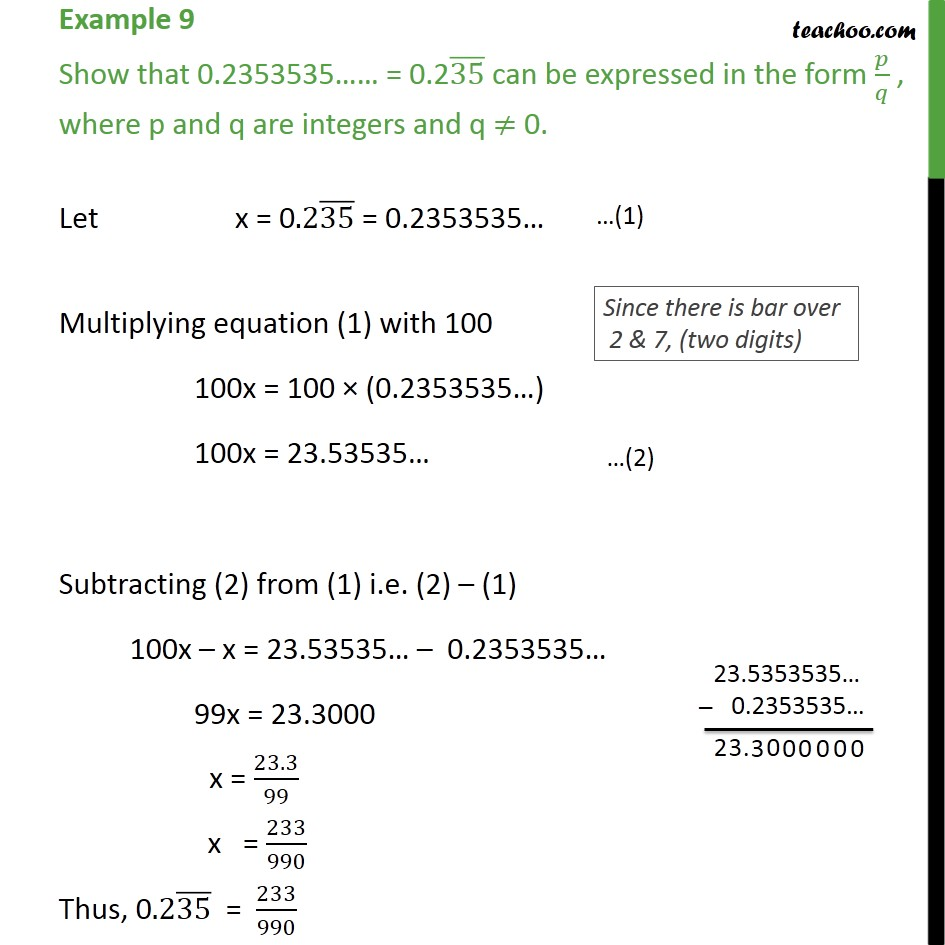Examples

Chapter 1 Class 9 Number Systems
Serial order wiseGet live Maths 1-on-1 Classs - Class 6 to 12

### Transcript

Example 9 Show that 0.2353535…… = 0.2(35) ̅ can be expressed in the form 𝑝/𝑞 , where p and q are integers and q ≠ 0. Let x = 0.2(35) ̅ = 0.2353535… Multiplying equation (1) with 100 100x = 100 × (0.2353535…) 100x = 23.53535… Subtracting (2) from (1) i.e. (2) – (1) 100x – x = 23.53535… – 0.2353535… 99x = 23.3000 x = 23.3/99 x = 233/990 Thus, 0.2(35) ̅ = 233/990